Courses

# SSC CHSL Mock Test -5

## 100 Questions MCQ Test SSC CHSL Mock Test Series | SSC CHSL Mock Test -5

Description
This mock test of SSC CHSL Mock Test -5 for SSC helps you for every SSC entrance exam. This contains 100 Multiple Choice Questions for SSC SSC CHSL Mock Test -5 (mcq) to study with solutions a complete question bank. The solved questions answers in this SSC CHSL Mock Test -5 quiz give you a good mix of easy questions and tough questions. SSC students definitely take this SSC CHSL Mock Test -5 exercise for a better result in the exam. You can find other SSC CHSL Mock Test -5 extra questions, long questions & short questions for SSC on EduRev as well by searching above.
QUESTION: 1

### In the following question, some part of the sentence may have errors. Find out which part of the sentence has an error and select the appropriate option. If a sentence is free from error, select 'No Error'.  Q. I am not sure if they (1)/ are aware in the new programme (2)/ implemented by the Government. (3)/ No error (4)

Solution:

The error lies in part 2 of the sentence as the preposition 'in' is incorrect after the word 'aware' and must be replaced with 'of' as someone is aware 'of' something. 'aware of' means 'to have knowledge of something.' Option 2 is thus the correct answer.

QUESTION: 2

### In the following question, some part of the sentence may have errors. Find out which part of the sentence has an error and select the appropriate option. If a sentence is free from error, select 'No Error'.  Q. I am disappointed to (1)/ see that neither of the (2)/ websites have any offers. (3)/ No error (4)

Solution:

Neither and either are singular and require singular verbs even though they seem to be referring, in a sense, to two things. Thus the plural verb 'have' is incorrect and must be replaced with the singular verb 'has. The error lies in part 3 of the sentence.

QUESTION: 3

### In the following question, out of the four alternatives, select the alternative which will improve the bracketed part of the sentence. In case no improvement is needed, select "no improvement". Q. The world economy is changing at a (rapidly paced) and new developments are revolutionizing the education sector.

Solution:

The noun 'pace' is correct here and the adjective 'rapid' is correct here as it acts upon the noun. Option 2 is thus the correct answer. The adverb 'rapidly' and the other options are incorrect here.

QUESTION: 4

In the following question, out of the four alternatives, select the alternative which will improve the bracketed part of the sentence. In case no improvement is needed, select "no improvement".

Q. The new educational systems in (era of) globalization are global.

Solution:

The article 'the' is mandatory as the sentence is referring to something definite. The correct preposition here is 'of' as 'era' is followed with of'. Option 1 is thus the correct answer. The other options are grammatically incorrect.

QUESTION: 5

A sentence has been given in Active/Passive Voice. Out of the four given alternatives, select the one which best expresses the same sentence in Passive/Active Voice.

Q. My teacher instructed me to write the essay again.

Solution:

The object is written before the subject in passive voice.

Object +verb +subject

The correct tense here is simple past tense 'was instructed' as the tense in active voice is simple past. Option 4 is thus the correct answer.

QUESTION: 6

They were not happy with the dog in such a condition.

Solution:

The subject is written before the object in active voice.

subject +verb +object

The correctly structured sentence is option 3 as 'did not make' is correct here.Option 3 is thus the correct answer

QUESTION: 7

A sentence has been given in Direct/Indirect Speech. Out of the four given alternatives, select the one which best expresses the same sentence in Indirect/ Direct Speech.

Q. Reema said,"I want to sleep now."

Solution:

The words in indirect speech must be followed by the word 'that' if that is not a question. The correct tense is simple past as the tense in direct speech is simple present. The word 'now' becomes 'then' in indirect speech. Option 2 is the correct answer.

QUESTION: 8

A sentence has been given in Direct/Indirect Speech. Out of the four given alternatives, select the one which best expresses the same sentence in Indirect/ Direct Speech.

Q. The teacher said,"Have you come prepared?"

Solution:

Since it is a question the word 'if' must be used to frame the sentence correctly in indirect speech. The correct tense is past perfect as the tense in direct speech is present perfect. Option 3 is thus the correct answer.

QUESTION: 9

Fill in the blank with the correct word.

Q. The company was transformed and the Ward _________ a vital role in bringing this about

Solution:

The correct tense here is past perfect as the sentence talks about an activity which was completed before another activity. Generally past perfect tense is used to refer to an activity which had taken place before another one.

Eg: She had completed her task when the bell rang. When I went to their house they had completed dinner.

Option 3 is thus the correct answer.

QUESTION: 10

In the following question, out of the four alternatives, select the alternative which best expresses the meaning of the idiom/phrase.

Mums the word.

Solution:

The correct option is option 3.
To understand the meaning better, we can use it in a sentence.

For example:
He gave me a signal to mum my words while we were standing in the principal's office.

QUESTION: 11

In the following question, out of the given four alternatives, select the alternative which best expresses the meaning of the Idiom/Phrase.

Rip up old sores

Solution:

The phrase ‘rip up old sores’ means to revive a quarrel which was almost forgotten. Therefore the correct answer is option 3.

QUESTION: 12

In the question below, a part of the sentence is highlighted. Below are given alternatives to the underlined part which may improve the sentence. Choose the correct alternative. In case no improvement is needed, choose "No improvement."

Q. If the man had a little more gluttony, then he wouldn't be so afraid of failing.

Solution:

The word 'gluttony' means 'habitual greed or excess in eating' and does not convey any meaning here. The meanings of the words are as follows:

Rapaciousness ⇒ Having or showing a strong or excessive desire to acquire money or possess things

Gumption ⇒ confidence and guts

Synergy ⇒ The interaction or cooperation of two or more organizations, substances, or other agents to produce a combined effect greater than the sum of their separate effects

Thus option 2 is the correct answer.

QUESTION: 13

In the question below, a part of the sentence is highlighted. Below are given alternatives to the underlined part which may improve the sentence. Choose the correct alternative. In case no improvement is needed, choose "No improvement."

Q. The moot point is that we are not left with any other option.

Solution:

The word 'moot' means 'critical or debatable' and fits here correctly and needs no improvement. The meanings of the words are:
Voracious ⇒ Insatiable
Grotesque ⇒ Extremely ugly and strange
Thus option 4 is the correct answer.

QUESTION: 14

The sentence given with blank is to be filled with an appropriate word. Four alternatives are suggested for each question. Choose the correct alternative and click the button corresponding to it.

Q. The speaker kept _______ into subjects unrelated to his speech.

Solution:

To digest is to take in and process. To divest is to reduce or remove one's interests in something. To disgrace is to humiliate someone. To digress is to move away from the main topic of something; which is the most apt solution here. Therefore, the correct answer is option 4.

QUESTION: 15

The sentence given with blank is to be filled with an appropriate word. Four alternatives are suggested for each question. Choose the correct alternative and click the button corresponding to it.

Q. The king had given orders to punish the _______ that went against the Church.

Solution:

The word 'heretics' means people that behaved against the authority of King or the Church.

Gangsters are criminals or people committing unlawful activities. Naysayers are people that are against one's actions or opinions. 'Families' is not an apt option here. Therefore, the correct answer is option 1.

QUESTION: 16

In the following question, out of the four alternatives, choose the word which best expresses the meaning of the given word and click the button corresponding to it.

Sagacious

Solution:

Correct option - 4

Firstly, let's find out the meaning of 'sagacious' :- having or showing keen mental discernment and good judgement; wise or shrewd.

Now, find the meaning of following words :-

Sleuth - a detective.

Bounty - a sum paid for killing or capturing a person or animal.

Clearly, option 4 i.e. shrewd gives the most similar meaning of word 'sagacious'.

Hence, option 4 is the correct answer.

QUESTION: 17

In the following question, out of the four alternatives, choose the word which best expresses the meaning of the given word and click the button corresponding to it.

Concatenation

Solution:

Correct option - 2

Firstly, let's find out the meaning of 'Concatenation':- a series of interconnected things; sequence.

Now, find the meaning of following words:-

Aghast - filled with horror or shock.

Integration - the action or process of integrating; interconnection.

Reinstated - to restore someone or something to their former position or state.

Elegance - the quality of being pleasingly ingenious and simple; neatness.

Clearly, option 2 i.e. integration gives the most similar meaning of word "concatenation".

Hence, option 2 is the correct answer.

QUESTION: 18

In the following question, out of the four alternatives, choose the word which is opposite in meaning to the given word and click the button corresponding to it.

Tautology

Solution:

Correct option - 3

Firstly, let's find out the meaning of 'Tautology' -a phrase or expression in which the same thing is said twice in different words; repetition; periphrase; wordiness.

Now, find the meaning of following words:-

Conciseness - Exactness; clear; straight.

Ramification -a complex or unwelcome consequence of an action or event; result.

Clearly, option 3 i.e. conciseness is the most opposite in meaning to given word.

So, option 3 is the correct answer.

QUESTION: 19

In the following question, out of the four alternatives, choose the word which is opposite in meaning to the given word and click the button corresponding to it.

Innuendo

Solution:

Correct option - 3

Firstly, let's find out the meaning of 'Innuendo' - an indirect intimation about a person or thing, especially in derogatory nature without any proof; implication; overtone.

Now, find the meanings of following words:-

Fable - a short story, typically with animals as characters, conveying a moral.

Fugitive - a person who has escaped from captivity or is in hiding.

Evidence - proof; confirmation; verification; documentation.

Here, evidence gives the most opposite meaning to given word 'innuendo'.

Hence, option 3 is the correct answer.

QUESTION: 20

To waste time, money, or energy on trifling matters.

Solution:

Correct option - 3

Let's find out the meanings of following words:-

Pent-up - emotions, energy, etc.that are unable to be expressed or released.

Strew -scatter or spread things untidily over a surface or area.

Fritter -waste of time, money, or energy on trifling matters.

Afloat - to float.

So, option 3 i.e. fritter is the word that best substitutes the given phrase.

QUESTION: 21

In the following question, out of the four alternatives, select the alternative which is the best substitute of the phrase.

Q. A detailed plan for a journey, especially a list of places to visit.

Solution:

Correct option - 2

Let's find out the meaning of following words :-

Pristine - clean and fresh as if new; spotless.

Itinerary - a travel document recording a route or journey.

Zephyr  - a soft gentle breeze.

Fora - plural of forum.

So, option 2 i.e. itinerary is the word that best substitutes the given phrase.

QUESTION: 22

In the following question, four words are given out of which one word is incorrectly spelt. Select the incorrectly spelt word.

Solution:

Correct option - 2
Here, option 2 gives the incorrect spelling. Its correct spelling is grandeur. It means splendour and impressiveness, especially of appearance or style; high rank or social importance.

Now, find the meaning of following options :-

Gratuitous - Given or obtained without charge or payment; free; voluntary.

Glutton - A person who eats and drinks excessively or voraciously.

Thus, option 2 is the correct answer.

QUESTION: 23

In the following question, four words are given out of which one word is incorrectly spelt. Select the incorrectly spelt word.

Solution:

Correct option - 3
Here, option 3 is incorrectly spelt. Its correct spelling is emancipation. It means the fact or process of being set free from legal, social, or political restrictions; liberation.

Now, find the meaning of following words :-
Exasperation - The state of being irritated; extreme annoyance.
Clearly, option 3 is the correct answer.

QUESTION: 24

In the following question, the first and the last parts of the sentences have been numbered 1 and 6. The rest of the sentence is split into four parts and named P, Q, R and S. These four parts are not given in their proper order. Read the following sentence and find out which of the four combinations is correct. Then mark the correct answer from the options given below.

1. A beautiful landscape with
P. but fill us with
Q. full of green vegetation
R. our attention
S. will not just attract
6. infinite satisfaction.

Solution:

Looking at Q and S, the starter of the sentence has a conjunction with at the end which can better take an adjective full of green vegetationbut not a verb will. Thus, options 1 and 3 are eliminated. Now, in continuation with QS, attract is a verb which should take an object our attention with it. Thus, on this ground option can be removed.Thus, correct answer is 4.

CORRECT SENTENCE- A beautiful landscape with full of green vegetation will not just attract our attention but fill us with infinite satisfaction.

QUESTION: 25

In the following question, the first and the last parts of the sentences have been numbered 1 and 6. The rest of the sentence is split into four parts and named P, Q, R and S. These four parts are not given in their proper order. Read the following sentence and find out which of the four combinations is correct. Then mark the correct answer from the options given below.

1. Once upon a time
P. and enjoyed a lot
Q. to a near by hill station
R. but was very sorry
S. I went for a week’s holiday
6. when the week was over.

Solution:

The starter of the sentence once upon a time should have some noun/pronoun in its continuation. Thus, options 1 and 3 are eliminated. Now, looking at other two, the sentence can not have phrase as "but was very sorry to a near by hill stations" (RQ). This is grammatically incorrect. Thus, on this ground option 4 is eliminated. Hence, correct answer is 2.

CORRECT SENTENCE- Once upon a time I went for a week’s holiday to a near by hill station and enjoyed a lot but was very sorry when the week was over.

QUESTION: 26

In the following question, select the odd word from the given alternatives.

Solution:

Tie, necklace and bow tie are the things which we wear around the neck whereas we wear ring in the fingers.
Hence, ‘Ring’ is the correct answer.

QUESTION: 27

In the following question, select the number which can be placed at the sign of question mark (?) from the given alternatives.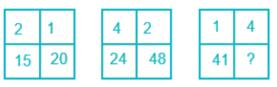Solution:

The pattern follow in each square is as follows:
151 + 22 + 13 = 15 + 4 + 1 = 20
241 + 42 + 2= 24 + 16 + 8 = 48
Similarly,
411 + 12 + 4= 41 + 1 + 64 = 106
Hence, 106 is the correct answer.

QUESTION: 28

If the following words will arrange in the sequence as they appear in the dictionary, then what will be the sequence as per the dictionary order?
1. ONLINE
2. ONCE
3. ONLY
4. ONSET
5. ONION

Solution:

The sequence followed is:
2. ONCE
5. ONION
1. ONLINE
3. ONLY
4. ONSET
Hence, ‘25134’ is the correct answer.

QUESTION: 29

If a mirror is placed on the line AB, then which of the answer figure is the correct image of the given figure?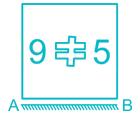Solution:

If a mirror is placed along AB line, then only the top and bottom part of a given image will be interchanged in the mirror image while everything else will remain same.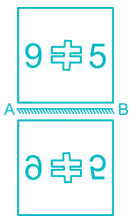Hence, option 3 is the correct mirror image of the given question.

QUESTION: 30

P, Q and R have a partnership firm. P’s profit share is 2/3rd of the Q’s profit share and R’s share is 1/2 of the Q’s share. If total revenue is Rs. 43296 and total expense is Rs. 9899, then what will be the profit share of R?

Solution:

Net Profit = Total Revenue – Total Expense = Rs. 43296 – Rs. 9899 = Rs. 33397

Let, the profit share of Q is Rs. x.
→ P’s profit share = Rs. 2x/3
→ R’s profit share = Rs. x/2

Thus we have,
Net Profit = P’s profit share + Q’s profit share + R’s profit share
33397 = 2x/3 + x + x/2
33397 = (4x + 6x + 3x)/6
33397 = 13x/6
x = (33397 × 6)/13
= 15414
R’s share = 15414/2 = 7707
Hence, Rs. 7707 is the correct answer.

QUESTION: 31

Among the four answer figures which can be formed from the cut-out pieces given below.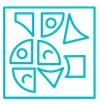Solution: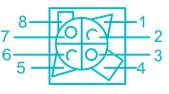Hence, the answer is option 2.

QUESTION: 32

In the following question, select the odd letter from the given alternatives.

Solution:

The pattern followed here is,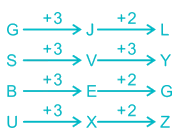Hence, SVY is the odd one.

QUESTION: 33

A series is given with one term missing. Select the correct alternative from the given ones that will complete the series.

249, 270, 261, 282, 273, ?

Solution:

The pattern followed here is,
249 + 21 = 270,
270 - 9 = 261,
261 + 21 = 282,
282 - 9 = 273,
Similarly,
273 + 21 = 294
Hence, 294 is the correct answer.

QUESTION: 34

Find out the figure which best represents the relationship Cornea, Intestine and Eye.

Solution:

Cornea lies inside the eye and intestine is different from eye and cornea. So, below figure represents the best relation among these three.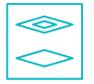Figure given in option 4 represents the best relation among these three.
Hence, option 4 is the correct one.

QUESTION: 35

In the following question below are given three statements followed by three conclusions. Taking the given statements to be true even if they seem to be at variance from commonly known facts, read all the conclusions and then decide which of the given conclusion logically follows the given statements.

Statements:
I. Some Mobile are Pen.
II. All Pen are Paper.
III. Some Paper are Table.

Conclusions:
I. Some Pen are Table
II. Some Paper are Mobile
III. No Table being a Pen is a possibility

Solution:

Consider the following least possible Venn diagram,Conclusions:
I. Some Pen are Table → False (It is possible but not definite).
II. Some Paper are Mobile → True
III. No Table being a Pen is a possibility → True (Possibility is true).
Hence, only conclusion II and III follow.

QUESTION: 36

From the given answer figures, select the one in which the question figure is hidden/embedded?Solution:

On close observation, we find that the problem figure is embedded in figure (3) as shown below: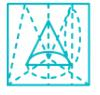Hence, figure 1 is the correct answer.

QUESTION: 37

Which answer figure will complete the pattern in the question figure?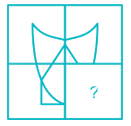Solution: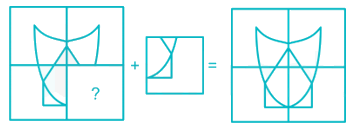Hence, option 1 completes the pattern in question figure.

QUESTION: 38

In the following question, select the related word from the given alternatives

Jeweller : Jewellery ∷ Cartographer : ?

Solution:

Jeweller is the person who makes jewellery. Similarly, cartographer is the person who makes maps.
Hence, ‘Map’ is the correct answer.

QUESTION: 39

If ‘red’ is called ‘green’, ‘green’ is called ‘yellow’, ‘yellow’ is called ‘navy blue’ and ‘navy blue’ is called ‘pink’, then what is the colour of Ashoka chakra in the Indian flag?

Solution:

Since, the colour of the Ashoka chakra is navy blue and here, navy blue is called pink.

Hence, pink is the correct answer.

QUESTION: 40

In a certain code language, "FINDER" is written as "GEDNIX". How is "WALNUT" written in that code language?

Solution:

The pattern used here is,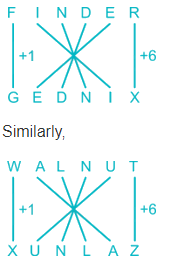Hence, XUNLAZ is the correct answer.

QUESTION: 41

If “A @ B means, A is sister of B”, “A \$ B means, A is a daughter of B”, “A # B means A is a wife of B”, “A % B means A is a brother of B”, then how Q is related to U in the expression “Q \$ T # A % P @ U”?

Solution:

From the given expression “Q \$ T # A % P @ U”, we get:
Q \$ T means Q is a daughter of T.
T # A means T is a wife of A.
A % P means A is a brother of P.
P @ U means P is a sister of U.
Thus, using the following notations, we can draw the family tree: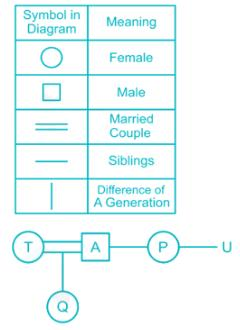So, Q is a niece of U.
Hence, ‘Niece’ is the correct answer.

QUESTION: 42

If you will arrange the following options, it will give you a meaningful word. Select the odd word from the given alternatives.

Solution:

On arranging the given alternatives, we get:
1) LOYOGOICS → SOCIOLOGY
2) EGYOHAPRG → GEOGRAPHY
3) GYTTORRENMOI → TRIGONOMETRY
4) THOIYRS → HISTORY

Sociology, Geography and history are the subjects of the social studies whereas trigonometry is the subject of mathematics.
Hence, ‘GYTTORRENMOI’ is the odd one.

QUESTION: 43

In the following question, select the related letter from the given alternatives.

SWORD : UURTB : : WORDS : ?

Solution:

The pattern followed here is,
In ‘SWORD : UURTB’,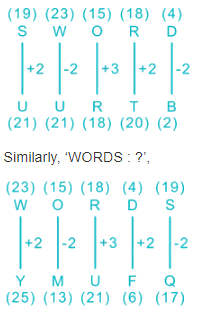Hence, ‘YMUFQ’ is the correct answer.

QUESTION: 44

How many quadrilaterals are there in the given figure?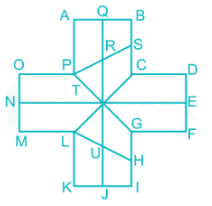Solution:

Quadrilaterals (means 4 - sided figure) in the given figure are as follows:Here, quadrilaterals are: LKUJ, LKJT, LKIH, JIGT, JIHU, LHGT, GTUH, GFET, DCTE, TCSR, SBQR, QAPR, TCSP, ABSP, TCBQ, TQAP, PONT, TNML.
Hence, there are 18 quadrilaterals in the given figure.

QUESTION: 45

In a row, B is standing at 26th position from the last of the row and A is standing 51st from the front of the row. If from the front, B is standing ahead of A and there are 7 persons standing between them, then find the total no. of persons in a row?

Solution:

B’s position from the last = 26th
A’s position from the front = 51st
B is standing ahead of A. So, B’s position from the front = 51 - 7 - 1 = 43rd position
Total no. of persons = B’s position from the front + No. of persons after B
= 43 + 25 = 68
Hence, 68 is the correct answer.

QUESTION: 46

A mixed series is given with one term missing. Select the correct alternative from the given ones that will complete the series.

5D, 7G, 10I, 14L, ?

Solution:

The pattern followed here is,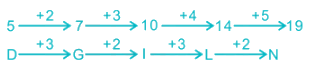Hence, 19N is the correct answer.

QUESTION: 47

Select the correct combination of mathematical signs to replace ∗ signs and to balance the given equation.

27 ∗ 2 ∗ 270 ∗ 5

Solution:

Let us check each option,
1) 27 ÷ 2 = 270 × 5 ⇒ 13.5 ≠ 1350, which is false.
2) 27 = 2 × 270 ÷ 5 ⇒ 27 ≠ 108, which is false.
3) 27 ÷ 2 × 270 = 5 ⇒ 3645 ≠ 108, which is false.
4) 27 × 2 = 270 ÷ 5 ⇒ 54 = 54, which is true.
Hence, ‘×, =, ÷’ is correct sequence.

QUESTION: 48

If 1739 \$ 47 @ 7 = 30 and 30 @ 464 \$ 16 = 1, then 53 @ 1127 \$ 23 = ?

Solution:

Here, ‘\$’ means ‘ ÷ ’ and ‘@’ means ‘ - ’.
The pattern followed is as follows:
1739 \$ 47 @ 7 = 1739 ÷ 47 - 7 = 37 - 7 = 30
30 @ 464 \$ 16 = 30 – 464 ÷ 16 = 30 - 29 = 1
Similarly,
53 @ 1127 \$ 23 = 53 – 1127 ÷ 23 = 53 - 49 = 4
Hence, 4 is the correct answer.

QUESTION: 49

In the following question, select the word which cannot be formed using the letters of the given word.

WORSHIPPED

Solution:

Let’s check each option,
1) SHOWER → Can be formed as WORSHIPPED.
2) POWDER → Can be formed as WORSHIPPED.
3) SIPPER → Can be formed as WORSHIPPED.
4) TOWER → Cannot be formed as there is no ‘T’ in WORSHIPPED.
Hence, TOWER can’t be formed from word WORSHIPPED.

QUESTION: 50

In the following question, select the odd number pair from the given alternatives.

Solution:

The pattern followed here is,
486 – 18 → 486 ÷ 18 = 27
297 – 11 → 297 ÷ 11 = 27
784 – 28 → 784 ÷ 28 = 28
432 – 16 → 432 ÷ 16 = 27
Hence, ‘784 – 28’ is the odd one.

QUESTION: 51

Find the value of x in the following expression:

[(52)x + 2 × (5- x)4 = (5)x – 5]

Solution:

Given,
⇒ (52)x + 2 × (5- x)4 = (5)x – 5
⇒ (5)(2x + 4 – 4x) = (5)x – 5
⇒ (5)(4 – 2x) = (5)x – 5
⇒ 4 – 2x = x – 5
∴ x = 3

QUESTION: 52

A shopkeeper marked a bag at 35% above its cost price. If he sold it at a discount of 12%, find his profit percentage.

Solution:

Let the cost price of the bag be Rs. ‘x’
Marked price = (100 + 35)% of x = 1.35x
Discount = 12% of 1.35x = 0.12 × 1.35x = 0.162x
Selling price = Marked price – discount = 1.35x – 0.162x = 1.188x
Profit earned = selling price – cost price = 1.188x – x = 0.188x
∴ Profit percentage = (0.188x/x) × 100 = 18.8%

QUESTION: 53

Which of the following belong to the set of non-positive integers?

Solution:

Zero is neither positive nor negative. Hence, it is included in both the sets of non-negative and non-positive integers.

QUESTION: 54

P and Q can together do a work in 6 days. If P can do the work alone in 18 days, then P is:

Solution:

P’s 1 day work = 1/18
(P + Q)’s 1 day work = 1/6
Q’s 1 day work = 1/6 – 1/18 = 1/9
Hence, Q can alone do the work in 9 days
∴ P is half as efficient as Q

QUESTION: 55

A man can swim upstream at a speed of 4 km/hr and downstream at 8 km/hr. In how much time will he cover a distance of 3 km in still water?

Solution:

Upstream speed = 4 km/hr
Downstream speed = 10 km/hr
Speed in still water = ½ × (downstream speed + upstream speed) = ½ × (8 + 4) = 6 km/hr
∴ Required time = 3/6 = 0.5 hrs = 30 min

QUESTION: 56

How much time will a 150 m long train take to cross a 180 m long platform, if it is running at a speed of 45 km/hr?

Solution:

Speed of train = 45 km/hr = 45 × (5/18) = 12.5 m/sec
Distance to cover = 150 + 180 = 330 m
∴ Time taken by train to cross the platform = 330/12.5 = 26.4 sec

QUESTION: 57

The sum and the difference of two numbers is 120 and 10 respectively. Find the sum of their squares.

Solution:

Let the numbers be ‘a’ and ‘b’
Hence, (a + b) = 120 and (a – b) = 10
As we know, (a + b)2 + (a – b)2 = 2(a2 + b2)
⇒ Sum of their squares = (a2 + b2) = [(a + b)2 + (a – b)2]/2
∴ Sum of their squares = [(120)2 + (10)2]/2 = (14400 + 100)/2 = 14500/2 = 7250

QUESTION: 58

The actual radius and height of a cylindrical vessel is 2.14 cm and 10.15 cm respectively. But they were expressed nearly to two significant places. What percentage error occurs while computing the volume of the cylinder? Use π = 22/7.

Solution:

Actual volume of cylinder = πr2h = 22/7 × 2.14 × 2.14 × 10.15 = 146.089 cm3
Computed volume of cylinder = πr2h = 22/7 × 2.1 × 2.1 × 10 = 138.6 cm3
Error in volume = 146.089 – 138.6 = 7.489 cm3
∴ Required percentage = (7.489/146.089) × 100 = 5.126%

QUESTION: 59

The sum of a number and 4 times its reciprocal is 4. Find the number.

Solution:

Let the number be ‘x’
⇒ x + 4(1/x) = 4
⇒ x2 + 4 = 4x
⇒ x2 – 4x + 4 = 0
⇒ (x – 2)2 = 0
⇒ x = 2
∴ The required number is 2

QUESTION: 60

If the average of X and Y is 200, the average of Y and Z is 238.5 and that of X and Z is 152.5. Find the average of X, Y and Z.

Solution:

Given, (X + Y)/2 = 200
⇒ X + Y = 400 …(1)
Also, (Y + Z)/2 = 238.5
⇒ Y + Z = 477 …(2)
Also, (X + Z)/2 = 152.5
⇒ X + Z = 305 …(3)
⇒ 2(X + Y + Z) = 400 + 477 + 305
⇒ X + Y + Z = 1182/2 = 591
⇒ (X + Y + Z)/3 = 591/3 = 197
∴ The required average is 197

QUESTION: 61

A dishonest shopkeeper has faulty weights of 1 kg and 500 g which measures 940 g and 470 g respectively. What is his percentage profit?

Solution:

Original weights = 1000 + 500 = 1500 g
Faulty weights = 940 + 470 = 1410 g
Error = 1500 – 1410 = 90 g
∴ Required profit percentage = [error/(original value – error)] × 100 = (90/1410) × 100 = 6.38%

QUESTION: 62

A car is moving at a speed of 50 km/hr. A truck which is 10 km away from the car is moving towards opposite to it at a speed of 70 km/hr. After how much time will they meet?

Solution:

As the car and truck are moving in opposite directions, their relative speed = sum of their individual speeds = 50 + 70 = 120 km/hr
Distance to cover = 10 km
∴ Time after they meet = 10/120 = 1/12 hr = 60/12 = 5 min

QUESTION: 63

When a number is divided by 208, the remainder is 3. Find the remainder, when the same number is divided by 13.

Solution:

Let the dividend and the quotient be ‘x’ and ‘q’ respectively
⇒ x = 208q + 3
⇒ x = (13 × 16)q + 3
⇒ x = 13(16q) + 3
∴ The remainder is 3

QUESTION: 64

How many squares of side 5 cm can be cut from a rectangular sheet of dimensions 25 cm × 15 cm?

Solution:

Area of rectangular sheet = 25 × 15 = 375 cm2
Area of square = 5 × 5 = 25 cm2
∴ No. of squares that can be cut = 375/25 = 15

QUESTION: 65

If (7x – 3y) ∶ (4x + y) = 5 ∶ 4, then find the ratio x ∶ y.

Solution:

Given,
⇒ (7x – 3y) ∶ (4x + y) = 5 ∶ 4
⇒ [7(x/y) – 3] / [4(x/y) + 1] = 5/4
⇒ 28(x/y) – 12 = 20(x/y) + 5
⇒ x/y = 17/8
∴ The required ratio is 17 ∶ 8

QUESTION: 66

A ladder is resting against a wall at a height of 50 m. Find the length (in m) of the ladder, if the angle of elevation with the ladder is 15°.

Solution: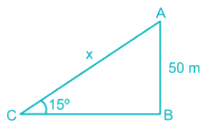Let length of the ladder be x m.
Given,
Height of the wall h = 50 m
In right angled △ABC,
⇒ sin15° = AB/AC
⇒ (√3 – 1) /2√2 = 50/x
⇒ x = {50/ (√3 – 1)} × 2√2 × [(√3 + 1) / (√3 + 1)]
⇒ x = 100 × √2(√3 + 1) / (3 – 1)
⇒ X = 50√2(√3 + 1)
∴ Length of the ladder is 50√2(√3 + 1) m.

QUESTION: 67

A man borrowed Rs. 12500 at a simple interest of 8% per annum for 1 year and 73 days. How much amount did he pay to clear his debt?

Solution:

Time = 1 + 73/365 = 1 + 1/5 = 6/5 yrs.
Now,
Simple interest = (Principal × rate × time)/100 = (12500 × 8 × 6/5)/100 = Rs. 1200
∴ Amount paid to clear debt = 12500 + 1200 = Rs. 13700

QUESTION: 68

A question paper comprises of 150 questions out of which 60 questions are of technical field. From the remaining questions, 40% are from English, 40% are from reasoning and the remaining from GK. If 80% of the technical, 50% of the English, 50% of the reasoning and 50% of the GK questions are compulsory, what percentage of the total questions is compulsory to attempt?

Solution:

No. of technical questions = 60
No. of English questions = 40% of (150 – 60) = 0.4 × 90 = 36
No. of reasoning questions = 40% of (150 – 60) = 0.4 × 90 = 36
No. of GK questions = 150 – 60 – 36 – 36 = 18
Compulsory technical questions = 0.8 × 60 = 48
Compulsory English questions = 0.5 × 36 = 18
Compulsory reasoning questions = 0.5 × 36 = 18
Compulsory GK questions = 0.5 × 18 = 9
Total compulsory questions = 48 + 18 + 18 + 9 = 93
∴ Required percentage = (93/150) × 100 = 62%

QUESTION: 69

Find the smallest fraction.

Solution:

Solving the fractions and converting to decimal,
⇒ (12/5 + 8/3) = 76/15 = 5.067
⇒ (11/4 + 7/2) = 25/4 = 6.25
⇒ (10/3 + 9/4) = 67/12 = 5.583
⇒ (9/2 + 6/5) = 57/10 = 5.7
∴ The smallest fraction is (12/5 + 8/3)

QUESTION: 70

A man mixed 20 kg of brick powder costing Rs. 20 per kg with 80 kg of chili powder costing Rs. 100 per kg and sold the mixture for Rs. 110 per kg. Find his profit percentage.

Solution:

Cost price of 100 kg mixture = 20 × 20 + 80 × 100 = 400 + 8000 = Rs. 8400
Selling price of 100 kg mixture = 100 × 110 = Rs. 11000
Profit earned = 11000 – 8400 = Rs. 2600
∴ Profit percentage = (2600/8400) × 100 = 30.95 ≅ 31%

QUESTION: 71

Find the approximate value of: (90.195 ÷ 2.942 × 121.012 ÷ 3.963)

Solution:

Approximating the terms as,
⇒ 90.195 = 90
⇒ 2.942 = 3
⇒ 121.012 = 120
⇒ 3.963 = 4
Hence, the equation is approximated to,
⇒ 90 ÷ 3 × 120 ÷ 4
⇒ 30 × 30
⇒ 900

QUESTION: 72

Directions: The given table shows the mass number and the atomic number of various elements. In an atom, the number of protons (p) is equal to the atomic number and the number of neutrons (n) is the difference between the mass number and the atomic number. Study the table and answer the questions that follow.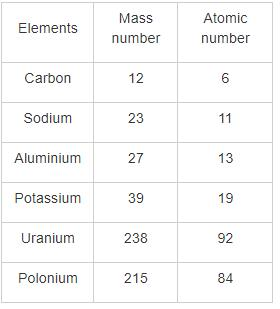Q. Find the neutron to proton (n/p) ratio of Uranium.

Solution:

Mass no. of Uranium = 238
Atomic no. of Uranium = 92
No. of protons = p = 92
No. of neutrons = n = 238 – 92 = 146
∴ Required ratio = n/p = 146/92 = 1.59

QUESTION: 73

Directions: The given table shows the mass number and the atomic number of various elements. In an atom, the number of protons (p) is equal to the atomic number and the number of neutrons (n) is the difference between the mass number and the atomic number. Study the table and answer the questions that follow.Q. What is the difference between the number of neutrons in Potassium and Aluminium?

Solution:

No. of neutrons in Potassium = 39 – 19 = 20
No. of neutrons in Aluminium = 27 – 13 = 14
∴ Required difference = 20 – 14 = 6

QUESTION: 74

Directions: The given table shows the mass number and the atomic number of various elements. In an atom, the number of protons (p) is equal to the atomic number and the number of neutrons (n) is the difference between the mass number and the atomic number. Study the table and answer the questions that follow.Q. What is the average number of protons in Carbon, Sodium and Polonium?

Solution:

No. of protons in Carbon = 6
No. of protons in Sodium = 11
No. of protons in Polonium = 84
∴ Required average = (6 + 11 + 84)/3 = 101/3 = 33.67

QUESTION: 75

Directions: The given table shows the mass number and the atomic number of various elements. In an atom, the number of protons (p) is equal to the atomic number and the number of neutrons (n) is the difference between the mass number and the atomic number. Study the table and answer the questions that follow.Q. What is the difference between the n/p ratio of Sodium and Potassium?

Solution:

n/p ratio of Sodium = (23 – 11)/11 = 12/11 = 1.09
n/p ratio of Potassium = (39 – 19)/19 = 20/19 = 1.05
∴ Required difference = 1.09 – 1.05 = 0.04

QUESTION: 76

Aipan is a traditional folk art of _________.

Solution:
• Aipan is a traditional folk art of Uttarakhand.
• It is one of the traditional forms of rangoli practiced there.
• Aipan designs in Uttarakhand are mainly drawn at the places of worship or at the front courtyard or entry door of the house.
QUESTION: 77

Who is the author of the book 'Indian Fiscal Federalism'?

Solution:
• N.K. Singh, Chairman, 15th Finance Commission, launched the book 'Indian Fiscal Federalism.
• The book is written by Dr. Y. V. Reddy, former Governor of RBI and Chairman of the 14th Finance Commission of India along with Dr. G. R. Reddy, Advisor (Finance) to the Government of Telangana.
QUESTION: 78

Which among the following films won the Critics Best Film award at the 64th Filmfare Awards held at Jio Garden, Mumbai?

Solution:
• The 64th Filmfare awards were held at Jio Garden, Mumbai on 23 March 2019.
• Raazi won the Best Film award and Alia Bhatt received the Best Actress award for Raazi.
• Ranveer Singh won the Critics Best Actor award for Padmaavat.
• Ranbir Kapoor won the Best Actor award for Sanju.
• Andhadhun won the Critics Best Film award.
QUESTION: 79

When is the World Tuberculosis Day observed every year?

Solution:
• World Tuberculosis Day is observed every year on 24 March.
• The aim of the day is to raise public awareness about the devastating health, social and economic consequences of TB, and to step up efforts to end the global TB epidemic.
• The theme of World TB Day 2019 - ‘It’s time’.
• The World Health Organization (WHO) has launched a joint initiative 'Find. Treat. All. #EndTB'.
QUESTION: 80

Who has been appointed as the Deputy Chief Minister of Goa in March 2019?

Solution:
• Goa Minister for Tourism and Sports, Manohar Ajgaonkar has been appointed as the Deputy Chief Minister of the state.
• Ajgaonkar had left the Maharashtrawadi Gomantak Party (MGP) to join the BJP.
• Newly-inducted BJP MLA Deepak Pauskar was also inducted into Chief Minister Pramod Sawant's cabinet.
QUESTION: 81

Which one of the following is a Peacetime Gallantry Award?
1. Shaurya Chakra
2. Vir Chakra
3. Kirti Chakra
4. Ashok Chakra Award

Solution: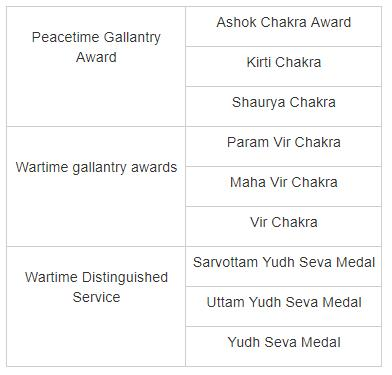QUESTION: 82

In 'Pradhan Mantri JI-VAN Yojana'. What does N stand for ?

Solution:
• The Union Cabinet approved the 'Pradhan Mantri JI-VAN Yojana' (Jaiv Indhan- Vatavaran Anukool fasal Awashesh Nivaran) on 28 February 2019.
• The aim of the scheme is to provide financial support to Integrated Bioethanol Projects using lignocellulosic biomass and other renewable feedstock.
• The yojana will be supported with total financial outlay of Rs.1969.50 crore for 5 years.
QUESTION: 83

The Executive Board of the International Olympic Committee (IOC) has recommended adding which of the following to the 2024 Paris Olympics?
1. Breakdancing
2. Skateboarding
3. Surfing

Solution:
• The Executive Board of the International Olympic Committee (IOC) recommended adding breakdancing, skateboarding, sport climbing and surfing to the 2024 Paris Olympics.
• The addition of these 4 sports to the Paris medal program offers the opportunity to connect with the young generation.
• All 4 sports will be assessed in terms of how they are managed, the integrity of competitions and judging.
QUESTION: 84

Who among the following is the chairman of the 15th Finance Commission of India?

Solution:
• Nand Kishore Singh(NK Singh) is the chairman of the 15th Finance Commission of India and this will give recommendations for the period of 2020-2025.
• Finance Commissions are formed by the President of India under the provisions of Article 280 of the Indian Constitution.
• The main terms of reference of finance commission include the definition of the financial relationship between the central government and the state governments.
• The first such commission was formed in 1951 with KC Neogy being the chairman of that commission.
QUESTION: 85

Economic growth is usually coupled with ________.

Solution:
• Inflation is a good indicator of economic growth because whenever there is economic growth, the wealth of the people of the country also increases.
• Due to increase in wealth, the spending increases. As the spending increases, the demand increases leading to increase in price causing inflation.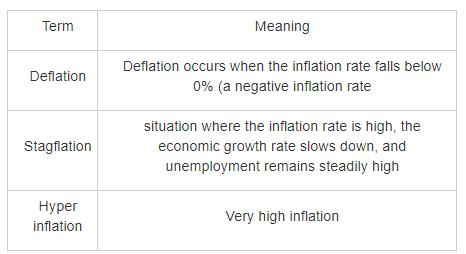QUESTION: 86

The older Rs. 20 Currency Note has which among the following on its reverse side?

Solution:
• The Rs. 20 Currency Note has the portrait of Mahatma Gandhi on the obverse side with the signature of the RBI governor.
• On the reverse side, the currency note has the motif of the Mount Harriet in the Andaman and Nicobar Islands.
QUESTION: 87

The Convention on Biological Diversity came into force in the year ________.

Solution:
• The Convention on Biological Diversity came into force in the year 1993 whereas the same was opened for signature in 1992 in Rio Earth Summit.
• The main aim is to devise national strategies to protect biodiversity in the world.
• This protocol has two components - Cartagena Protocol and Nagoya Protocol.
• The Cartagena Protocol is regarding the movements of the Living Modified Organisms whereas the Nagoya Protocol is about the fair and equitable sharing of genetic resources based benefits.
QUESTION: 88

Economic Cooperation Organization is headquartered in which of the following cities?​

Solution:
• Economic Cooperation Organization (ECO) is headquartered in Tehran.
• It is a Eurasian economic and political intergovernmental organization, founded by leaders of Turkey, Iran and Pakistan in the year 1984.
• World Bank and IMF are headquartered in Washington D.C.
• Shanghai is the headquarters of New Development Bank also called the BRICS bank.
• Asian Development Bank is headquartered in Manila.
QUESTION: 89

Which of the following vitamins is responsible for blood clotting?

Solution:

Vitamin K is responsible for the coagulation of blood also known as blood clotting.

• Vitamin K helps in the process of blood coagulation as it acts as a cofactor for the enzymes which are required for maintaining the blood clotting factors, namely Protein C, S, and factors VII, IX,and X.
• Vitamin K is found in the following foods: Green leafy vegetables, such as kale, spinach, turnip greens, collards, Swiss chard, mustard greens, parsley, romaine, and green leaf lettuce.
QUESTION: 90

White Rust of Crucifers is due to

Solution:

White Rust of Crucifers is a plant disease caused by fungi albugo candida and cystopus candida.

QUESTION: 91

What is 1 Horse Power (HP)?

Solution:
• 1 Horse Power = 746 Watts
• Horse Power is a unit which expresses the power or the rate of work done.
• There are two types of Horse Power unit
1. Mechanical Horse power = 746 Watts
2. Metric Horse Power = 735.5 Watts
QUESTION: 92

Who gave the plum-pudding model for an atom?

Solution:
• In 1898, J. J. Thomson gave the plum-pudding model for the structure of an atom.
• According to this model, an atom is in the form of a sphere in which the positive charges are uniformly distributed and the electrons are embedded in these positive charges similar to plums in a pudding, hence, the name, plum-pudding model.
QUESTION: 93

Which of the following is the deepest lake in the world?

Solution: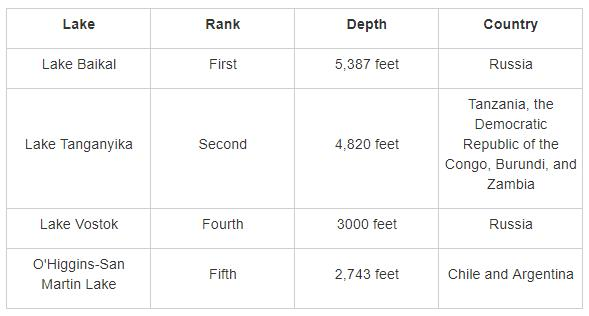QUESTION: 94

Given below are climatic zones and major crops grown in them. Identify the INCORRECT match.

Solution:
• Mainly crops like millets, sorghum are grown and also short cycle of beans and groundnuts can be grown in semi-arid zone.
• Sub-humid zone have crops like millets, sorghum, sweet potatoes, mango, etc. are grown here.
• Moist sub-humid zone have crops like maize, sugarcane, banana, pineapple, etc. are grown here.
• Humid zone have crops like rubber, maize, sugarcane, rice, banana, etc. are grown here.
QUESTION: 95

Which of the following two water bodies are connected by the Yucatan Strait?

Solution:
• The Yucatan Strait connects the Gulf of Mexico and the Caribbean Sea.
• When two large water bodies get naturally connected by a narrow waterway, then that waterway is termed as a strait.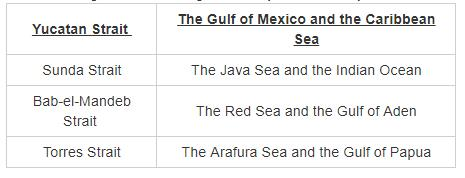QUESTION: 96

Who gave Vallabhai Patel the title of ‘Sardar’?

Solution:

It was by the women of Bardoli, during the struggle and after the Indian National Congress victory in Bardoli Satyagraha, that Patel first began to be referred to as Sardar.

QUESTION: 97

Where did Gautam Buddha finally attain enlightenment?

Solution:
• Gautam Buddha attained enlightenment and became a Buddha in the Mahabodhi Temple at Bodh Gaya while he was meditating under the Bodhi tree.
• This place is now a well-known pilgrimage site.
QUESTION: 98

What can be a maximum gap between two sessions of Parliament in India?

Solution:

The maximum gap between two sessions of Parliament in India should not be more than 6 months. That means, the Parliament should meet at least twice a year.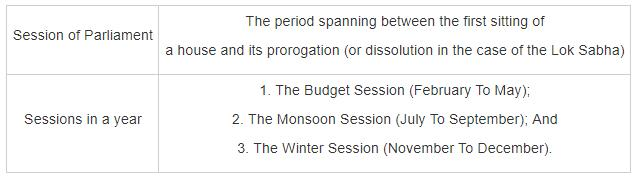QUESTION: 99

The idea of Ombudsman is taken from which of the following countries?

Solution:
• Ombudsman(Lokpal) is an official who is charged with representing the interests of the public by investigating and addressing complaints of maladministration or violation of rights.
• Swedish Parliamentary started Ombudsman by the Instrument of Government of 1809, to safeguard the rights of its citizens.
QUESTION: 100

How many Fundamental Duties are given in Indian Constitution?

Solution:
• There are 11 Fundamental duties given in Indian Constitution.
• The fundamental duties are contained in Article 51A.
• Fundamental duties were added by 42nd and 86th Constitutional Amendment acts.